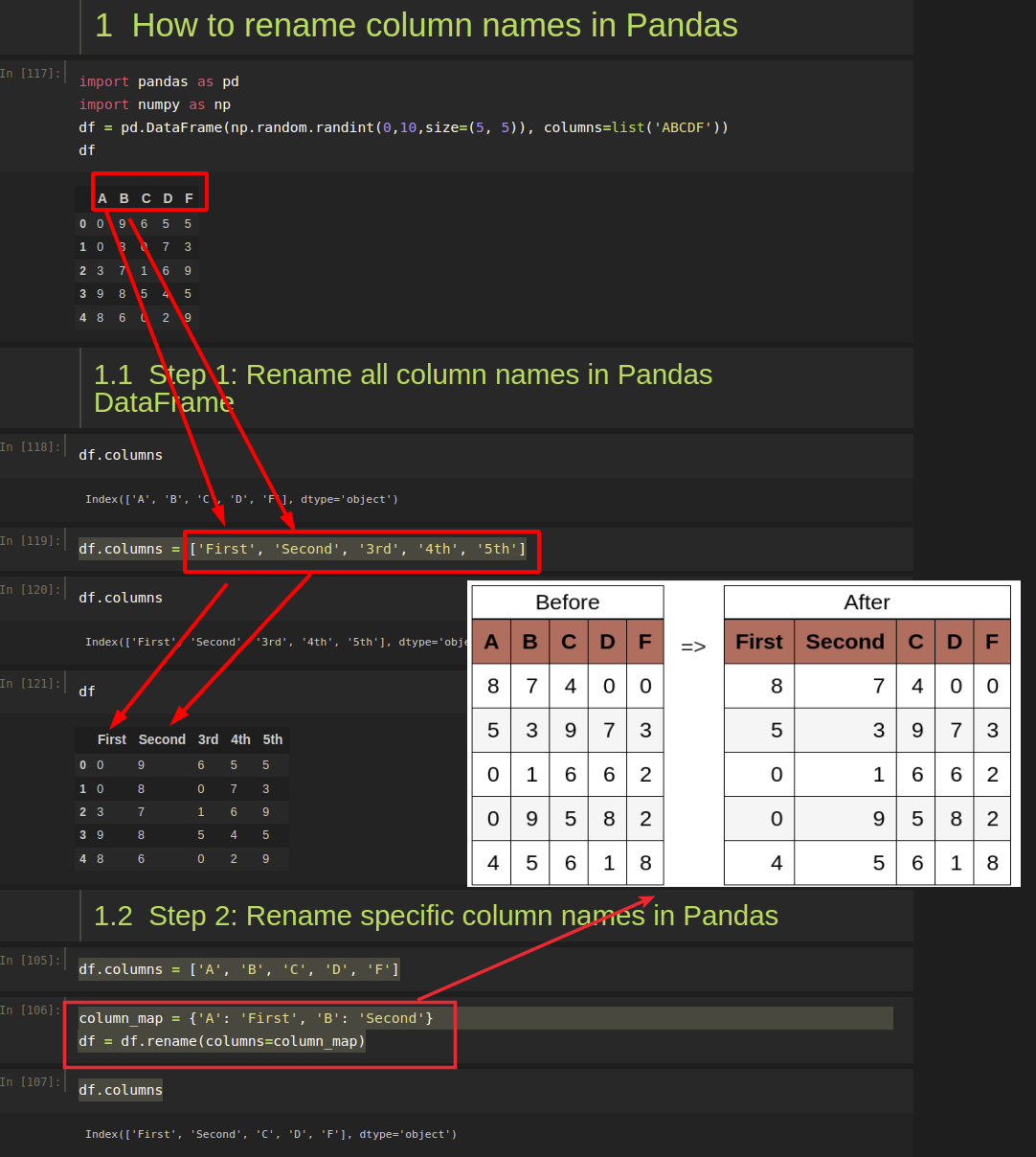In this short guide, I'll show you how to rename column names in Pandas DataFrame.

(1) rename single column

``````df.rename(columns = {'\$b':'B'}, inplace = True)
``````

(2) rename multiple columns

``````column_map = {'A': 'First', 'B': 'Second'}
df = df.rename(columns=column_map)
``````

(3) rename multi-index columns

``````cols = pd.MultiIndex.from_tuples([(0, 1), (0, 2)])
df = pd.DataFrame([[1,2], [3,4]], columns=cols)
``````

(4) rename all columns

``````df.columns = ['a', 'b']
``````

In the next sections, I'll review the steps to apply the above syntax in practice and a few exceptional cases.

Let's say that you have the following DataFrame with random numbers generated by:

``````import pandas as pd
import numpy as np
df = pd.DataFrame(np.random.randint(0,10,size=(5, 5)), columns=list('ABCDF'))
``````

DataFrame:

A B C D F
0 4 8 9 0 1
1 5 8 6 1 0
2 7 9 8 1 1
3 6 8 3 8 9
4 6 0 2 8 8

If you like to understand more about how to create DataFrame with random numbers please check: How to Create a Pandas DataFrame of Random Integers

## Step 1: Rename all column names in Pandas DataFrame

Column names in Pandas DataFrame can be accessed by attribute: `.columns`:

``````df.columns
``````

result:

``````Index(['A', 'B', 'C', 'D', 'F'], dtype='object')
``````

The same attribute can be used to rename all columns in Pandas.

``````df.columns = ['First', 'Second', '3rd', '4th', '5th']
``````
``````df.columns
``````

result:

``````Index(['First', 'Second', '3rd', '4th', '5th'], dtype='object')
``````
× Pro Tip
To rename single column in Pandas use:
`df.rename(columns = {'\$b':'B'}, inplace = True)`

## Step 2: Rename specific column names in Pandas

If you like to rename specific columns in Pandas you can use method - `.rename`. Let's work with the first DataFrame with names - A, B etc.

To rename two columns - `A, B` to `First, Second` we can use the following code:

``````column_map = {'A': 'First', 'B': 'Second'}
df = df.rename(columns=column_map)
``````

will result in:

``````Index(['First', 'Second', 'C', 'D', 'F'], dtype='object')
``````

Note: If any of the column names are missing they will be skipped without any error or warning because of default parameter `errors='ignore'`
Note 2: Instead of syntax: `df = df.rename(columns=column_map)` you can use `df.rename(columns=column_map, inplace=False)`× Pro Tip
Method rename can take a function:
`df.rename(columns=lambda x: x.lstrip())`
× Warning
Don't forget to use inplace=True to make the renaming permanent!

## Step 3: Rename column names in Pandas with lambda

Sometimes you may like to replace a character or apply other functions to DataFrame columns. In this example we will change all columns names from upper to lowercase:

``````df = df.rename(columns=lambda x: x.lower())
``````

the result will be:

``````Index(['a', 'b', 'c', 'd', 'f'], dtype='object')
``````

This step is suitable for complex transformations and logic.

## Step 4: Rename column names in Pandas with str methods

You can apply str methods to Pandas columns. For example we can add extra character for each column name with a regex:

``````df.columns = df.columns.str.replace(r'(.*)', r'Column \1')
``````

Working with the original DataFrame will give us:

``````Index(['Column A', 'Column B', 'Column C', 'Column D', 'Column F'], dtype='object')
``````

## Step 5: Rename multi-level column names in DataFrame

Finally let's check how to rename columns when you have MultiIndex. Let's have a DataFrame like:

``````import pandas as pd

cols = pd.MultiIndex.from_tuples([(0, 1), (0, 2)])
df = pd.DataFrame([[1,2], [3,4]], columns=cols)
``````
0
1 2
0 1 2
1 3 4

If we check the column names we will get:

``````df.columns
``````
``````MultiIndex([(0, 1),
(0, 1)],
)
``````

Renaming of the MultiIndex columns can be done by:

``````df.columns = pd.MultiIndex.from_tuples([('A', 'B'), ('A', 'C')])
``````

and will result into:

A
B C
0 1 2
1 3 4